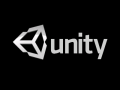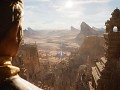• Register

## pkyrkos7

### Panos joined Jun 11, 2009

I am a programmer with a degree in Computer Science. I know plenty of programming languages including the most common such as C, C++, Java, Python, PHP, C# and others.
I have modded many games over the years mostly level designing with most important of them being Oblivion,Skyrim and some RTS games. I have experience with some game engines such as Game Maker, Unity, Unreal, Cryengine. I also have some experience with 3D modelling using Blender as well as some basic knowledge of Photoshop and video software.

My Blogs

#### Minesweeper In C

pkyrkos7 Blog

I've created a minesweeper game in C for a school project of mine and I decided to upload it here.Of course it needs to be compiled first since below there's only code.

``````#include<stdio.h>
#include<stdlib.h>
#include <ctype.h>
#include <math.h>
#include <time.h>

#define dimensions_small_table 15
int tile_choiceX,tile_choiceY,gbl_current_round=-1,gbl_mines=-1,tile_revealed_small_table[dimensions_small_table][dimensions_small_table],tile_checkZeros_small_table[dimensions_small_table][dimensions_small_table]; //global variables for small table
char gbl_Small_table[dimensions_small_table][dimensions_small_table],gbl_gameEnd='A';

int main()
{
int gotostart;
printf("Welcome to Minesweeper Game!\n");
do
{
gotostart=0;
printf("Press 1 to start playing!\n");
printf("Press 2 for information\n");
printf("Press 3 for legend\n");
printf("Press 4 to exit\n");
do
{
case '1':
SmallTableGame();
break;
case '2':
HelpFunction();
gotostart=1;
break;
case '3':
printf("\nLEGEND: *=tile not revealed,E=tile empty,B=tile has bomb\nNumber(1,...,8)=the number is equal to the amount of bombs at the nearby tiles\n\n");
gotostart=1;
break;
default:
return ProgramEnd();
}
if (gotostart!=1)
{
if (gbl_gameEnd=='L')
{
printf("\n\nYou found a mine!I am sorry but you lost!Do you want to try again?(Y=Yes,N=No)\n");
do
{ choice01=getch(); }
while (choice01!='N' &amp;&amp; choice01!='n' &amp;&amp; choice01!='Y' &amp;&amp; choice01!='y');
}
else if (gbl_gameEnd=='W')
{
printf("\n\n\nCongratulations!You have won the game!Want to play again?(Y=Yes,N=No)\n");
do
{ choice01=getch(); }
while (choice01!='N' &amp;&amp; choice01!='n' &amp;&amp; choice01!='Y' &amp;&amp; choice01!='y');
}
if (choice01=='Y' || choice01=='y')
gotostart=1;
}
} while (gotostart==1);
return ProgramEnd();
}

/*==================================This is the function used for the small table game==============================*/
int SmallTableGame()  //this table contains 225 tiles
{
//tile_choiceX and tile_choiceY have real number values --should be changed to use in arrays (real value-1)
int i,j,rand01,rand02,TotalTilesReveal,tilecounted[dimensions_small_table][dimensions_small_table],gotocoordinates;
//start up things below
gbl_gameEnd='A';//the value A means nothing.It could be anything apart from W or L
gbl_current_round=0;
TotalTilesReveal=0;
for (i=0;i<dimensions_small_table;i++)
{
for (j=0;j<dimensions_small_table;j++)
{
gbl_Small_table[i][j]=0;
tile_revealed_small_table[i][j]=0;
tile_checkZeros_small_table[i][j]=0;
tilecounted[i][j]=0;
}
}
/*---------------------------------------------*/
do
{
printf("Enter the amount of mines you want on your minefield(Max mines %.lf):",pow(dimensions_small_table,2)-1);
scanf("%d",&amp;gbl_mines);
} while (gbl_mines>=pow(dimensions_small_table,2) || gbl_mines<=0);
/*------------------*/
//this part of script will generate the minefield//
srand(time(0));//this line will make sure each time your run the program the first mine doesn't appear at the same place
for (i=0;i<gbl_mines;i++)
{
do
{
rand01=rand()%dimensions_small_table;
rand02=rand()%dimensions_small_table;
} while(gbl_Small_table[rand01][rand02]=='B');
gbl_Small_table[rand01][rand02]='B';
srand(time(0));//this line is very important DO NOT ERASE!Makes the minefield random
}
/*-------------------------------------------------*/
printf("\nGame has started!\n=====================\n");
do
{
putchar('\n');
if (gbl_current_round!=0)
{
if(gbl_Small_table[tile_choiceX-1][tile_choiceY-1]=='B')
gbl_gameEnd='L';
if (gbl_gameEnd!='L' &amp;&amp; gbl_gameEnd!='W' &amp;&amp; gbl_Small_table[tile_choiceX-1][tile_choiceY-1]!='B')
NearbyMineCheck(tile_choiceX,tile_choiceY);
for (i=0;i<dimensions_small_table;i++)
{
for (j=0;j<dimensions_small_table;j++)
{
if (tile_revealed_small_table[i][j]==1 &amp;&amp; tilecounted[i][j]==0 &amp;&amp; gbl_Small_table[i][j]!='B')
{
tilecounted[i][j]=1;
TotalTilesReveal++;
}
}
}
if ((TotalTilesReveal+gbl_mines)==pow(dimensions_small_table,2))
gbl_gameEnd='W';
}
gbl_current_round++;
printf("   ");
for (i=0;i<dimensions_small_table;i++)
{
if (i<9)
printf(" 0%d",i+1);
else
printf(" %d",i+1);
}
putchar('\n');
printf("   ");
for (i=0;i<dimensions_small_table*3;i++)
putchar('-');
for (i=0;i<dimensions_small_table;i++)
{
putchar('\n');
if (i<9)
printf("0%d| ",i+1);
else
printf("%d| ",i+1);
for (j=0;j<dimensions_small_table;j++)
{
if (gbl_gameEnd=='L')
{
if(gbl_Small_table[i][j]=='B' || tile_revealed_small_table[i][j]==1)
printf("%c  ",gbl_Small_table[i][j]);
else printf("*  ");
}
else
{
if (tile_revealed_small_table[i][j]==0)
printf("*  ");
else
printf("%c  ",gbl_Small_table[i][j]);
}
}
}
if (gbl_gameEnd!='L' &amp;&amp; gbl_gameEnd!='W')
{
do
{
gotocoordinates=0;
printf("\n\nEnter the coordinates of the tile you want to reveal.");
printf("\nX coordinate(row): ");
printf("\nY coordinate(column): ");
if (tile_revealed_small_table[tile_choiceX-1][tile_choiceY-1]==1)
{
printf("\nThe tile at %d,%d has already been revealed.Choose another.\n",tile_choiceX,tile_choiceY);
gotocoordinates=1;
}
} while (gotocoordinates==1);
}
} while (gbl_gameEnd!='L' &amp;&amp; gbl_gameEnd!='W');  //gbl_gameEnd will take the value L when the game is lost or W if it is won
return 0;
}

/*--------------------IMPORTANT FUNCTIONS BELOW-------------------------*/

//This function will check for nearby mines of the tile that was chosen//
NearbyMineCheck(int tempX,int tempY)
{
int i,j,tile_nearMinesCount,gotostart;
do
{
gotostart=0;
tile_nearMinesCount=NearbyMineCheck2(tempX,tempY);
gbl_Small_table[tempX-1][tempY-1]=tile_nearMinesCount+48; //48='0'
tile_revealed_small_table[tempX-1][tempY-1]=1;
tile_checkZeros_small_table[tempX-1][tempY-1]=1;
if (tile_nearMinesCount==0)
{
if (tempY>1)
NearbyMineCheck2(tempX,tempY-1);//left
if (tempY<dimensions_small_table)
NearbyMineCheck2(tempX,tempY+1);//right
if (tempX>1)
NearbyMineCheck2(tempX-1,tempY);//up
if (tempX<dimensions_small_table)
NearbyMineCheck2(tempX+1,tempY);//down
if (tempX>1 &amp;&amp; tempY>1)
NearbyMineCheck2(tempX-1,tempY-1);//up-left
if (tempX>1 &amp;&amp; tempY<dimensions_small_table)
NearbyMineCheck2(tempX-1,tempY+1);//up-right
if (tempX<dimensions_small_table &amp;&amp; tempY>1)
NearbyMineCheck2(tempX+1,tempY-1);//down-left
if (tempX<dimensions_small_table &amp;&amp; tempY<dimensions_small_table)
NearbyMineCheck2(tempX+1,tempY+1);//down-right
for (i=0;i<dimensions_small_table;i++)
{
for (j=0;j<dimensions_small_table;j++)
{
if (gbl_Small_table[i][j]=='0' &amp;&amp; tile_checkZeros_small_table[i][j]==0 &amp;&amp; tile_revealed_small_table[i][j]==1)
{
tempX=i+1;
tempY=j+1;
tile_checkZeros_small_table[i][j]=1;
gotostart=1;
}
if (gotostart==1)
break;
}
if (gotostart==1)
break;
}
}
} while (gotostart==1);
}

int NearbyMineCheck2(int tempX,int tempY) //This Function is part of NearbyMineCheck Function
{
int tile_nearMinesCount=0;
/*This function will reveal the nearby tiles*/
if (tempY>1  &amp;&amp; gbl_Small_table[tempX-1][tempY-2]=='B')
{tile_nearMinesCount++;}//left
if (tempY<dimensions_small_table &amp;&amp; gbl_Small_table[tempX-1][tempY]=='B')
{tile_nearMinesCount++;}//right
if (tempX>1 &amp;&amp; gbl_Small_table[tempX-2][tempY-1]=='B')
{tile_nearMinesCount++;}//up
if (tempX<dimensions_small_table &amp;&amp; gbl_Small_table[tempX][tempY-1]=='B')
{tile_nearMinesCount++;}//down
if (tempX>1 &amp;&amp; tempY>1)
{
if (gbl_Small_table[tempX-2][tempY-2]=='B')
{tile_nearMinesCount++;}//up-left
}
if (tempX>1 &amp;&amp; tempY<dimensions_small_table)
{
if (gbl_Small_table[tempX-2][tempY]=='B')
{tile_nearMinesCount++;}//up-right
}
if (tempX<dimensions_small_table &amp;&amp; tempY>1)
{
if (gbl_Small_table[tempX][tempY-2]=='B')
{tile_nearMinesCount++;}//down-left
}
if (tempX<dimensions_small_table &amp;&amp; tempY<dimensions_small_table)
{
if (gbl_Small_table[tempX][tempY]=='B')
{tile_nearMinesCount++;}//down-right
}
gbl_Small_table[tempX-1][tempY-1]=tile_nearMinesCount+48; //48='0'
tile_revealed_small_table[tempX-1][tempY-1]=1;
return tile_nearMinesCount;
}

{
int tile_choice,tile_choiceValid=-1;
do
{
scanf("%d",&amp;tile_choice);
if (tile_choice>=1 &amp;&amp; tile_choice<=dimensions_small_table)
tile_choiceValid=1; //Y is column
else
{
tile_choiceValid=0;
printf("\nY coordinate not within bounds.Must be within 1 and %d\n",dimensions_small_table);
}
} while (tile_choiceValid==0);
return tile_choice;
}

HelpFunction(void)
//This function is called when the user needs help
{
printf("\nThe player is initially presented with a grid of undifferentiated squares.Some randomly selected squares, unknown to the player, are designated to contain mines.The game is played by revealing squares of the grid.If a square containing a mine is revealed, the player loses the game. Otherwise, a digit is revealed in the square, indicating the number of adjacent squares (typically, out of the possible eight) that contain mines.\n\n");
}

int ProgramEnd(void)
{
system("pause");
return 0;
}

``````
Groups#### Indie Gamers

1,518 members Hobbies & Interests

For fans of indie games and hardcore indie gamers, no matter whether these indies are commercial or freeware/opensource. This hub used for many indie...#### Indie Devs

1,709 members Hobbies & Interests

A group dedicated to indie and standalone game development.#### Unity Games

1,856 members Fans & Clans

For all Unity developers and developers-to-be, both beginners and professionals!#### Unreal Engine Devs, Modders and Players

702 members Fans & Clans

Group for all fans, gamers, modders and developers of Unreal Engine 4 and Unreal Engine 5 games.#### Horrorfans

648 members Fans & Clans

For all fans of horror games. Those who wish to share your best horror experiences, connect news articles, share images, and find demo's.579 members Arts & Literature

For us folks who like to stay Free and use the Blender 3d program over every other costly options!#### Indie DB

2,694 members Official

Indie games are changing the world, one giant pixel at a time. With Indie DB we aim to support independent developers and their games, by providing them...#### Desura

10,068 members Entertainment & Press

Desura is a community driven digital distribution service for gamers, putting the best games, mods and downloadable content from developers at gamers...

#### Start a group >>

Comments  (0 - 10 of 21)

✯ I wish you a happy late new year! pkyrkos7 ( Moddb.com ) ✯

Hey happy even more late new Year!

hahahaha xD

Hey buddy anything new?

Pretty much the same :P

Hey! Thanks for watching The Isle! It's great to have support!

It has dinosaurs so I will definitely keep an eye on it mate!